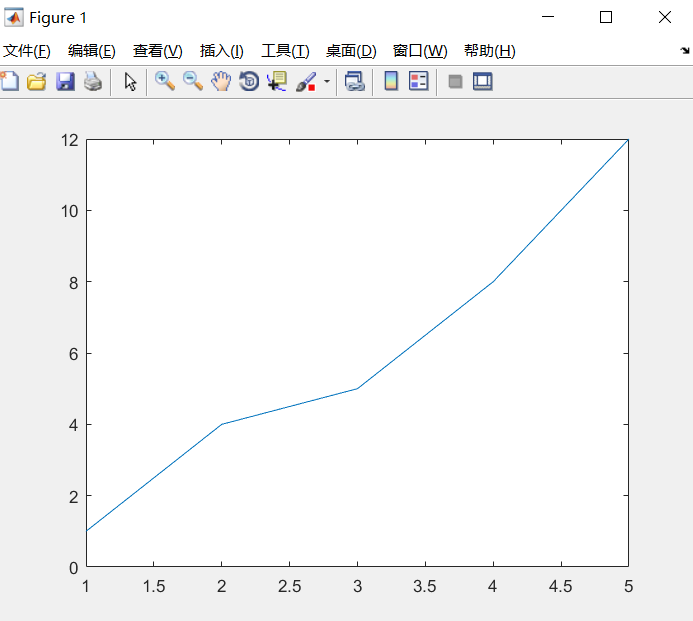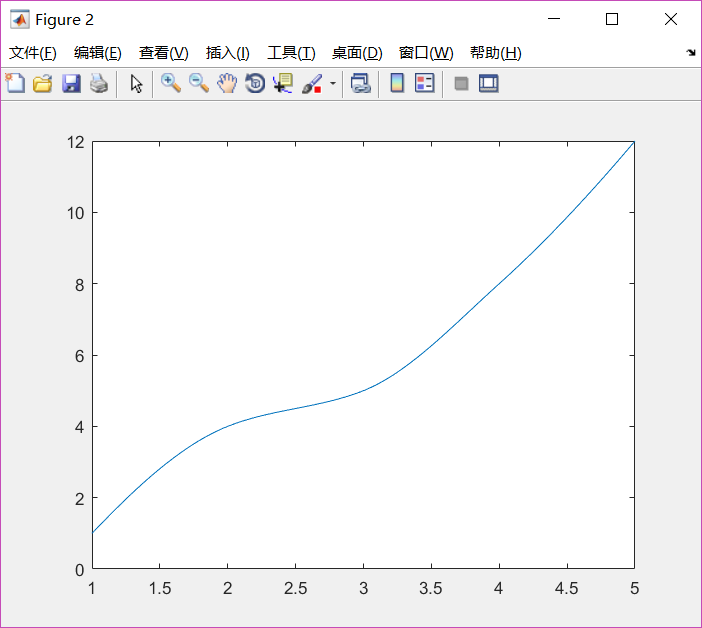• ## Matlab曲线平滑

万次阅读 2019-05-14 22:19:37
semilogy(x1,y1)%原来的折线 x2=linspace(min(x1),max(x1)); y2=interp1(x1,y1,x2,'cubic'); figure semilogy(x2,y2)%处理后的曲线 x=[1,2,3,4,5]; y=[1,4,5,8,12]; plot(x,y);...下面进行曲线平滑： x...
semilogy(x1,y1)%原来的折线
x2=linspace(min(x1),max(x1));
y2=interp1(x1,y1,x2,'cubic');
figure
semilogy(x2,y2)%处理后的曲线

x=[1,2,3,4,5];
y=[1,4,5,8,12];
plot(x,y);];

可以得到两个向量之间关系的折线图：下面进行曲线平滑：

x1=linspace(min(x),max(x));
y1=interp1(x,y,x1,'cubic');

plot(x1,y1);

曲线如下：注意！！

“interp1”函数式“一”不是“L”


展开全文• Matlab数据平滑处理主要用于对于MATLAB所描述的函数进行平滑处理
• ![图片说明]...怎样将图片中曲线进行平滑，尤其是对于红色蓝色交界处的平滑，我用 spcrv函数实现有点困难，有没有别的方法，或者spcrv函数使用有没有什么特殊的注意事项，哪位大神指导一下，万分感谢！图片
• ## matlab曲线平滑

千次阅读 2011-10-13 18:56:34
x=[0 0.1 0.16 0.27 0.41 0.48 0.59 0.8]; y=[5 9 70 118 100 17 0 5]; y1=[22.8 22.8 22.8 22.8 22.8 22.8 22.8 22.8]; values1=spcrv([[x(1) x x
 x=[0 0.1 0.16 0.27 0.41 0.48 0.59 0.8];
y=[5 9 70 118 100 17 0 5];
y1=[22.8 22.8 22.8 22.8 22.8 22.8 22.8 22.8];
values1=spcrv([[x(1) x x(end)];[y(1) y y(end)]],3,1000);
values2=spcrv([[x(1) x x(end)];[y1(1) y1 y1(end)]],3,1000);
plot(values1(1,:),values1(2,:),'r',values2(1,:),
values2(2,:),'b')

展开全文plot
• 发现了两个MATLAB自带的曲线平滑函数：smooth和spcrv 一、smooth 自己感觉：平滑效果还可以 1.语法 (1)函数 smooth（Y） smooth(Y,SPAN)%SPAN是滑动平均的点数，即“几点滑动平均” smooth(Y,SPAN,METHOD) （2）...
发现了两个MATLAB自带的曲线平滑函数：smooth和spcrv
一、smooth
自己感觉：平滑效果还可以
1.语法
(1)函数
smooth（Y）
smooth(Y,SPAN)%SPAN是滑动平均的点数，即“几点滑动平均”
smooth(Y,SPAN,METHOD)
（2）METHOD:
%loess:局部回归，加权的线性最小均方，2阶多项式模型
%rloess：'loess'的增强版，在回归过程中，给极端值分配的权重小。超出6倍绝对离差的极端值，权重为0
%'moving'：滑动平均（默认）。低通滤波器，滤波器系数=span的倒数
%'lowess':局部回归。线性最小均方加权，1阶多项式模型。
%'sgolay':Savitzky-Golay filter。
%‘rlowess’：‘lowess’的增强版。在回归过程中，给极端值分配的权重小。超出6倍绝对离差的极端值，权重为0
（3）注意
对增强版的算法而言，极端值的影响可以忽略
（4）例子1（来自MATLAB）
%-----------画出原始数据和平滑后的数据----
[xx,ind] = sort(x);%排序，ind-索引，xx-排序后的数据（从小到大）
subplot(2,1,1)
plot(xx,y(ind),'b.',xx,yy1(ind),'r-')
set(gca,'YLim',[-1.5 3.5])
legend('Original Data','Smoothed Data Using ''loess''','Location','NW')
subplot(2,1,2)
plot(xx,y(ind),'b.',xx,yy2(ind),'r-')
set(gca,'YLim',[-1.5 3.5])
legend('Original Data','Smoothed Data Using ''rloess''','Location','NW')
（5）例子2（来自MATLAB）
clc,clear
%1°滑动平均滤波器，5小时的span。平滑全部数据
c = smooth(count(:));
C1 = reshape(c,24,3);
%画图（原始数据，平滑后的数据）
subplot(3,1,1)
plot(count,':');
hold on
plot(C1,'-');
title('Smooth C1 (All Data)')
%2°相同的滤波器。分别对每列数据进行平缓。
C2 = zeros(24,3);
for I = 1:3,
C2(:,I) = smooth(count(:,I));
end
%画图（原始数据、平滑后的数据）
subplot(3,1,2)
plot(count,':');
hold on
plot(C2,'-');
title('Smooth C2 (Each Column)')
%3°画出两次滑动后的数据的差值
subplot(3,1,3)
plot(C2 - C1,'o-')
title('Difference C2 - C1')
二、spcrv
%20160518:spcrv函数
%目前可最做到，对原来的曲线进行平滑
%原理：增加点，平滑曲线
%（自己感觉）效果：可以平滑，但是：1°无法获得平滑后的曲线方程   2°平滑前后，曲线的起始点，y值大小不同
%% ------例子0----------------
points=[0 0 1 1 0 -1 -1 0 0;0 0 0 1 2 1 0 -1 -2];
figure(3)
plot(points(1,:),points(2,:),':');
values=spcrv(points,3);
hold on;plot(values(1,:),values(2,:));hold off
%% ------------例子1---------------------------
x=[0 0.1 0.16 0.27 0.41 0.48 0.59 0.8];
y=[5 9 70 118 100 17 0 5];
y1=[22.8 22.8 22.8 22.8 22.8 22.8 22.8 22.8];
values1=spcrv([[x(1) x x(end)];[y(1) y y(end)]],3,1000);
values2=spcrv([[x(1) x x(end)];[y1(1) y1 y1(end)]],3,1000);
plot(values1(1,:),values1(2,:),'r',values2(1,:),values2(2,:),'b')
%% ----------------------例子2-----------------
x1=[18.5931 19.2468 19.58193333 19.96586667 20.0985 20.2678 30.95973333];
y=[3.66 7.15 11.74 21.53 31.24 60.51 86.5]
values = spcrv([[x1(1) x1 x1(end)];[y(1) y y(end)]],3);
plot(values(1,:),values(2,:),'k');

展开全文• Matlab代码，输入一个Y输出smooth后的Y，适用如下 smoothY = smoothCurve(Y);
• ## MATLAB实现平滑处理

千次阅读 2020-10-16 20:23:33
一、五点三次平滑法 1.函数调用： 2.范例： clear all; clc; close all; xx=load('xnoisedata1.txt'); % 读入数据 time=xx(:,1); % 时间序列 x=xx(:,2); % 带噪数据 xmean=mean5_3(x,50); % 调用mean5_3函数,平滑...
一、五点三次平滑法
1.函数调用：2.范例：
clear all; clc; close all;
time=xx(:,1);                   % 时间序列
x=xx(:,2);                      % 带噪数据
xmean=mean5_3(x,50);            % 调用mean5_3函数,平滑数据
% 作图
subplot 211; plot(time,x,'k');
xlabel('时间/s'); ylabel('幅值')
title('原始数据'); xlim([0 max(time)]);
subplot 212; plot(time,xmean,'k');
xlabel('时间/s'); ylabel('幅值')
title('平滑处理后的数据'); xlim([0 max(time)]);
set(gcf,'color','w');二、smooth函数
1.函数调用：2.范例：
clear all; clc; close all;
k=1:500;                    % 产生一个从0到2*pi的向量，长为500
dn=2*pi/500;
x=cos((k-1)*dn);            % 产生一个周期余弦信号
[val,loc]=min(x);           % 求出余弦信号中的最小值幅值和位置
N=length(x);                % 数据长
ns=randn(1,N);              % 产生随机信号
y=x+ns(1:N)*0.1;            % 构成带噪信号
Err=var(x-y);               % 求x-y的方差
fprintf('%4d   %5.4f   %5.6f\n',loc,val,Err);

%y=y';                       % 转成列向量
z1=smooth(y,51,'moving');   % 'moving'平滑
Err1=var(x'-z1);            % 求x-z1的方差
[v1,k1]=min(z1);            % 求出平滑信号z1中的最小值幅值和位置
fprintf('1  %4d   %5.4f   %4d   %5.6f\n',k1,v1,abs(loc-k1),Err1);  % 显示

z2=smooth(y,51,'lowess');   % 'lowess'平滑
Err2=var(x'-z2);            % 求x-z2的方差
[v2,k2]=min(z2);            % 求出平滑信号z2中的最小值幅值和位置
fprintf('2  %4d   %5.4f   %4d   %5.6f\n',k2,v2,abs(loc-k2),Err2);

z3=smooth(y,51,'loess');    % 'loess'平滑
Err3=var(x'-z3);            % 求x-z3的方差
[v3,k3]=min(z3);            % 求出平滑信号z3中的最小值幅值和位置
fprintf('3  %4d   %5.4f   %4d   %5.6f\n',k3,v3,abs(loc-k3),Err3);

z4=smooth(y,51,'sgolay',3); % 'sgolay'平滑
Err4=var(x'-z4);            % 求x-z4的方差
[v4,k4]=min(z4);            % 求出平滑信号z4中的最小值幅值和位置
fprintf('4  %4d   %5.4f   %4d   %5.6f\n',k4,v4,abs(loc-k4),Err4);

z5=smooth(y,51,'rlowess');  % 'rlowess'平滑
Err5=var(x'-z5);            % 求x-z5的方差
[v5,k5]=min(z5);            % 求出平滑信号z5中的最小值幅值和位置
fprintf('5  %4d   %5.4f   %4d   %5.6f\n',k5,v5,abs(loc-k5),Err5);

z6=smooth(y,51,'rloess');   % 'rloess'平滑
Err6=var(x'-z6);            % 求x-z6的方差
[v6,k6]=min(z6);            % 求出平滑信号z6中的最小值幅值和位置
fprintf('6  %4d   %5.4f   %4d   %5.6f\n',k6,v6,abs(loc-k6),Err6);
% 作图
subplot 211; plot(k,x,'k');
grid; xlim([0 500]); title('一周期余弦信号')
xlabel('样点'); ylabel('幅值')
subplot 212; plot(k,y,'k'); %hold on
grid; axis([0 500 -1.5 1.5]); title('带噪一周期余弦信号')
xlabel('样点'); ylabel('幅值')
set(gcf,'color','w');

figure
subplot 321; plot(k,z1,'k'); title('moving法')
grid; axis([0 500 -1.5 1.5]); ylabel('幅值')
subplot 322; plot(k,z2,'k');  title('lowess法')
grid; axis([0 500 -1.5 1.5]); ylabel('幅值')
subplot 323; plot(k,z3,'k'); title('loess法')
grid; axis([0 500 -1.5 1.5]); ylabel('幅值')
subplot 324; plot(k,z4,'k'); title('sgolay法')
grid; axis([0 500 -1.5 1.5]); ylabel('幅值')
subplot 325; plot(k,z5,'k'); title('rlowess法')
grid; axis([0 500 -1.5 1.5]); xlabel('样点'); ylabel('幅值')
subplot 326; plot(k,z6,'k'); title('rloess法')
grid; axis([0 500 -1.5 1.5]); xlabel('样点'); ylabel('幅值')
set(gcf,'color','w');

无噪声信号与有噪声信号如下图所示：经过不同平滑后的信号如下图所示：以上结果不是固定不变的，这是因为每次叠加的随机数不用，这说明平滑处理的效果与叠加的噪声有关
三、Savitzky-Golay滤波器


展开全文• 基于matlab的代码，使用高斯平滑化方法处理图像，去噪声。
• x=[0 0.1 0.16 0.27 0.41 0.48 0.59 0.8]; y=[5 9 70 118 100 17 0 5]; y1=[22.8 22.8 22.8 22.8 22.8 22.8 22.8 22.8]; values1=spcrv([[x(1) x x(end)];[y(1) y y(end)]],3,1000); values2=spcrv([[x(1) x x(end)]...
• - PAGE PAGE 3 欢迎下载 MATLAB绘制平滑曲线 x=[0.1 0.16 0.27 0.41 0.48 0.59 0.8]; y=[8 70 118 100 9 0 5]; 以上是每一个X和Y对应的坐标请问如何编程能够绘制平滑曲线这个图形就像二次函数一样的 如果要在图中...
• https://blog.csdn.net/kunshao_07/article/details/80809631 需求 想要对两个列向量形成的曲线进行平滑处理。参考了@_Flamingo_的处理方法：https://blog.csdn.net/steelbasalt/article/details/48756143。两个列...
• 小编最近写文章的时候发现，训练网络，使用tensorboard进行可视化展示出来的精度曲线下载后生成csv或json类型的文件，小编尝试了使用各种...所以找了一段用matlab进行平滑曲线的程序。挂在上面。总的来说就是~~~~怕丢！
• 本文详细解释了从一张图片中识别文字的全过程，同时给出了MATLAB具体的代码实现。1. 文字识别过程此部分内容适合没有任何数字图像处理和编程经验的小白，请放心食用( ╯▽╰)。首先，我们知道一张数字图像是由无数个...
• MATLAB中绘制平滑曲线一般使用最小二乘法或者B样条插值。  最小二乘法实际上是函数拟合，可以得到目标函数（这里为多项式）的系数，对outliers相对不敏感，缺点是需要预先设置目标函数的阶数，且有时不容易找到最优...最小二乘法 B样条
• 1基本命令一般画函数或方程式之图形，都是先标上几个图形上的点，进而再将点连接即为函数图形，其点愈多图形越平滑MATLAB在简易2维画图(plot)，也是相同做法，必须先点出x 和y 坐标(离散数据)，再将这些点连接，...
• clear;x1=-0.05:0.03:0.2;x2=0:0.05:0.35;y1(:,1)=0;y1(:,2)=0;y1(:,3)=0.6;y1(:,4)=0.86;y1(:,5)=0.93;y1(:,6)=0.97;y1(:,7)=0.980;y1(:,8)=0.99;y1(:,9)=1.0;y2(:,1)=0;y2(:,2)=0.1;y2(:,3)=0.38;...y2(...
• ## matlab绘制平滑曲线

千次阅读 2013-09-27 22:49:09
以上是每一个X和Y对应的坐标，请问如何编程能够绘制平滑曲线，这个图形就像二次函数一样的 如果要在图中绘制一条直线加上y=70的直线，用不同颜色区分！   x=[0 0.1 0.16 0.27 0.41 0.48 0.59 0.8]; y=[5 9 70 ...
• 曲线拟合曲线拟合可以说在多种领域都有其用武之地，因为其主要的功能就是寻求平滑曲线来更好的表现带有噪声的测量数据。一般对于进行曲线拟合操作，都是先给定一部分采集的离散的数据点，在这些点的基础上建立数学...
• 原数据与软件处理后的数据相差并不大（如图所示），需要放大许多倍才能看出差别，对于结果呈现并不明显且影响不大，因此只在此说明，后面不再进行原数据与软件数据的比较。 原图 放大图 移动平均平滑 代码 ...
• S速度规划代码OpenRobotSL/TrajectoryPlanningS速度规划代码https://github.com/OpenRobotSL/TrajectoryPlanning​github.com基于S型曲线的连续多段曲线插补平滑过渡的规划算法（Matlab）_xuuyann-CSDN博客​blog....
• 需要预先安装matlab curving fitting tool。 直接上代码 x1 = [1.0 1.0 1.2 3.0 5.8 7.2 7.4]; y1 = [283 283 278 215 90 37 0]; x2 = [0.5 0.5 0.5 0.6 1.9 5.8 6.6 6.7]; y2 = [145 145 148 145 145 110 28 0];...
• 数据可视化数据可视化(Data Visualization)是指运用计算机图形学和图像处理技术，将数据转换为图形或图像在屏幕上显示出来，并进行交互处理的理论、方法和技术。它涉及计算机图形学、图像处理、计算机辅助设计、...
• 我试图检测图像中的弯曲输送... 曲线大致是在下图中手动绘制的(红色)： 我在matlab中没有找到用于Hough变换的代码或函数来检测这样的平滑曲线(例如,二次多项式：y = a * x ^ 2).任何其他解决方案也欢迎. 这是原始图片：
• ## MATLAB中数据平滑处理

万次阅读 多人点赞 2017-05-05 14:31:40
【例7.1-3】产生一列正弦波信号，加入噪声信号，然后调用medfilt1函数对加入噪声的正弦波进行滤波（平滑处理） % 产生一个从0到2*pi的向量，长度为500 >> t = linspace(0,2*pi,500)'; >> y = 100...数据
• 自然状态下，用plot画的是折线，而不是平滑曲线。 有两种方法可以画平滑曲线，第一种是拟合的方法，第二种是用spcrv，其实原理应该都一样就是插值。下面是源程序，大家可以根据需要自行选择，更改拟合的参数。 clc...
• 1、什么是平滑处理？  首先明白几个名词：  (1)噪声：图像噪声是指存在于图像数据中的不必要的或多余的干扰信息。 1.高斯噪声：高斯噪声是指它的概率密度函数服从高斯分布（即正态分布）的一类噪声。常见的高斯...滤波
• 今天写机械设计的带传动实验报告时，需要绘制滑动曲线ε-F和效率曲线η-F的关系曲线——正在学MATLAB的我内心狂喜，直接复制表格中的数据，掏出了plot函数。>> S=[0.348.55.50.364.64.80.380.630.780.63.34.......

# matlab曲线平滑处理matlab 订阅